# Difference between revisions of "Itô process"

$\newcommand{\Prob}{\mathsf{P}}$ $\newcommand{\Ex}{\mathsf{E}}$

A stochastic process with a stochastic differential. More precisely, a continuous stochastic process $X_t$ on a probability space $(\Omega, \mathcal{F}, \Prob)$ with a certain non-decreasing family $\{\mathcal F_t\}$ of $\sigma$-algebras ofis called an Itô process with respect to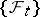if there exists processes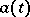and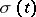(called the drift coefficient and the diffusion coefficient, respectively), measurable with respect tofor each, and a Wiener processwith respect to, such thatSuch processes are called after K. Itô , . One and the same process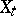can be an Itô process with respect to two different families. The corresponding stochastic differentials may differ substantially in this case. An Itô process is called a process of diffusion type (cf. also Diffusion process) if its drift coefficientand diffusion coefficientare, for each, measurable with respect to the-algebra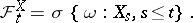Under certain, sufficiently general, conditions it is possible to represent an Itô process as a process of diffusion type, but, generally, with some new Wiener process (cf. ). If an Itô processis representable as a diffusion Itô process with some Wiener processand if the equation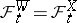is satisfied, then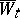is called the innovation process for.

Examples. Suppose that a certain Wiener process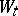,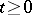, with respect tohas been given and suppose thatwhereis a normally-distributed random variable with meanand variancethat is measurable with respect to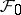.

The process, regarded with respect to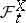, has stochastic differential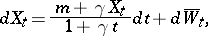in which the new Wiener process, defined byis an innovation process for.

How to Cite This Entry:
Itô process. Encyclopedia of Mathematics. URL: http://encyclopediaofmath.org/index.php?title=It%C3%B4_process&oldid=35534
This article was adapted from an original article by A.A. Novikov (originator), which appeared in Encyclopedia of Mathematics - ISBN 1402006098. See original article